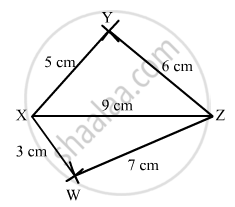Advertisement Remove all ads

# Construct a Quadrilateral Xyzw in Which Xy = 5 Cm, Yz = 6 Cm, Zw = 7 Cm, Wx = 3 Cm and Xz = 9 Cm. - Mathematics

Answer in Brief

Construct a quadrilateral XYZW in which XY = 5 cm, YZ = 6 cm, ZW = 7 cm, WX = 3 cm and XZ = 9 cm.

Advertisement Remove all ads

#### Solution

$\text { Steps of construction: }$

$\text { Step I: Draw XZ = 9 cm }$

$\text { Step II: With X as the centre and radius 5 cm, draw an arc above XZ } .$

$\text { Step III: With Z as the centre and radius 6 cm, draw an arc to intersect the arc drawn in Step II at Y above XZ }.$

$\text { Step IV: With Z as the centre and radius 7 cm, draw an arc below XZ .}$

$\text { Step V: With X as the centre and radius 3 cm, draw an arc to intersect the arc drawn in Step IV at W below XZ }.$

$\text { Step VI: Join XY, YZ, ZW and XW } .$

$\text { The quadrilateral WXYZ so obtained is the required quadrilateral } .$Is there an error in this question or solution?
Advertisement Remove all ads

#### APPEARS IN

RD Sharma Class 8 Maths
Chapter 18 Practical Geometry (Constructions)
Exercise 18.1 | Q 3 | Page 4
Advertisement Remove all ads

#### Video TutorialsVIEW ALL 

Advertisement Remove all ads
Share
Notifications

View all notifications

Forgot password?
Course